Investing 101 Lecture 4 Basic Portfolio Building Review

• Slides: 40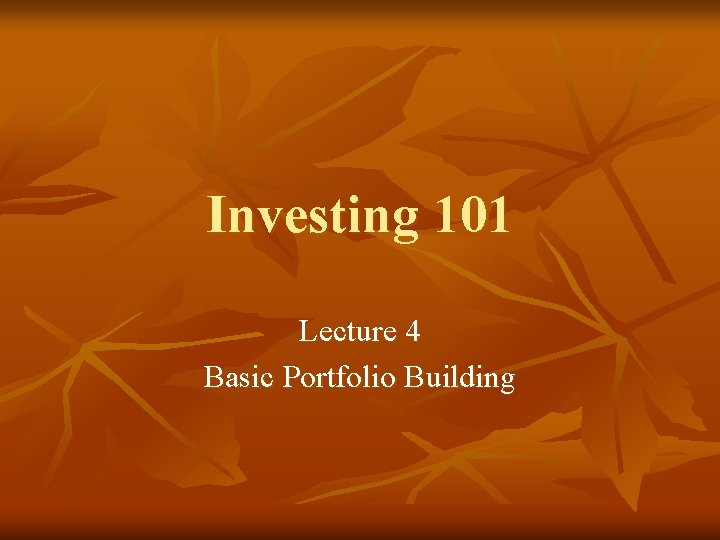Investing 101 Lecture 4 Basic Portfolio BuildingReview n Explain supply and demand? n What is GDP? n What are Consumer/Producer surplus? Reservation Price? n What is an interest rate? n What is an exchange rate?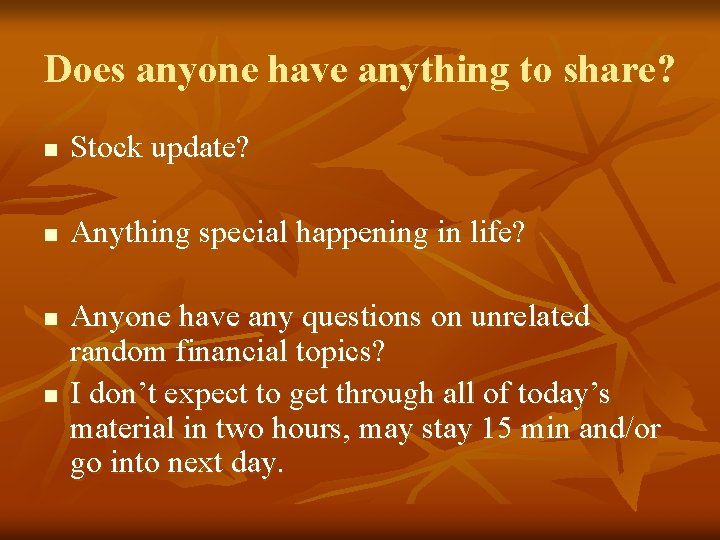Does anyone have anything to share? n Stock update? n Anything special happening in life? n n Anyone have any questions on unrelated random financial topics? I don’t expect to get through all of today’s material in two hours, may stay 15 min and/or go into next day.Main Teaching Points n Variance and standard deviation n Security market line (SML) n Capital allocation line (CAL) n Capital assets pricing model (CAPM) n Covariance and correlation n Diligent asset allocation n Pulling it all together.Warning n n n There are lots of slides in today’s class. Many of them are pictures and graphs. If you are a spatial mathematician you will want to pay close attention. There are some eqns you do NOT have to understand them, so if they confuse you do your best to block them out. Lets get started we have A LOT to learn and much to pull together.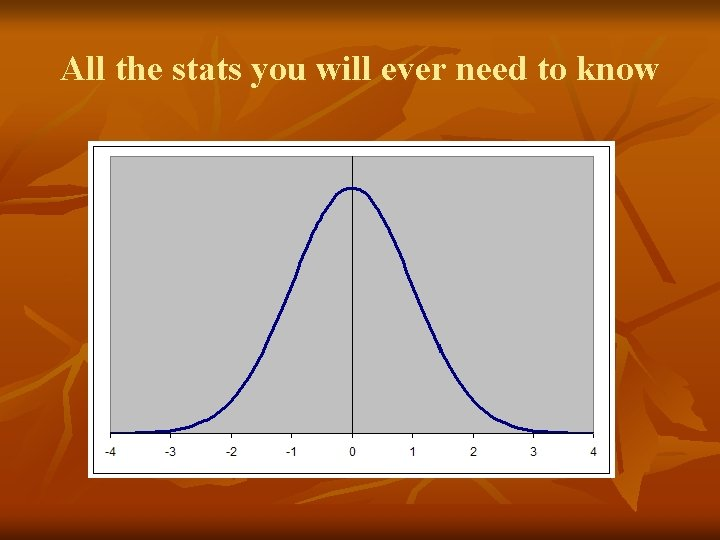All the stats you will ever need to knowEmpirical Rule n Has anyone heard of it? ? ? n 68 95 99 n The key to risk in stocks.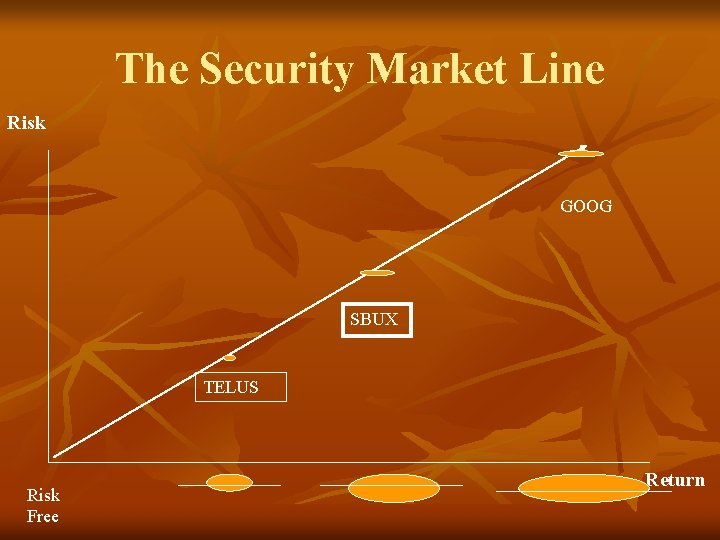The Security Market Line Risk GOOG SBUX TELUS Risk Free Return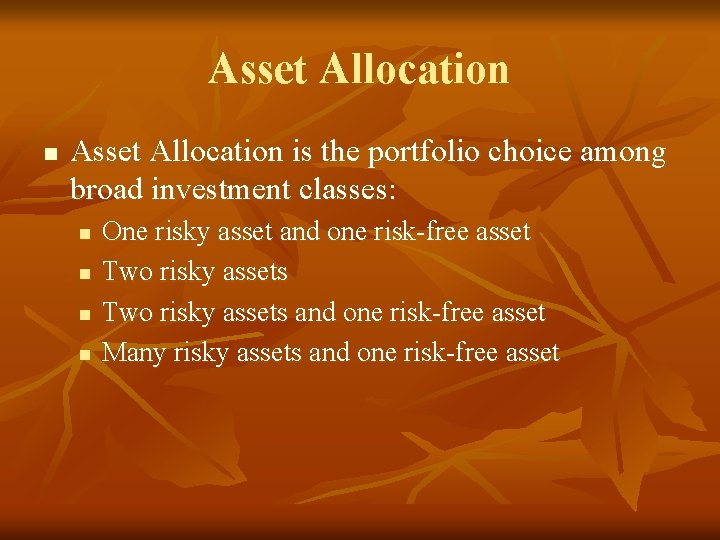Asset Allocation n Asset Allocation is the portfolio choice among broad investment classes: n n One risky asset and one risk-free asset Two risky assets and one risk-free asset Many risky assets and one risk-free asset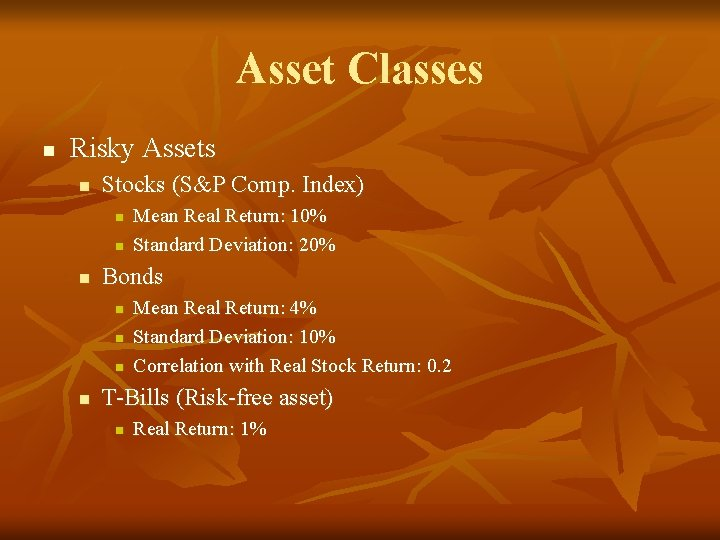Asset Classes n Risky Assets n Stocks (S&P Comp. Index) n n n Bonds n n Mean Real Return: 10% Standard Deviation: 20% Mean Real Return: 4% Standard Deviation: 10% Correlation with Real Stock Return: 0. 2 T-Bills (Risk-free asset) n Real Return: 1%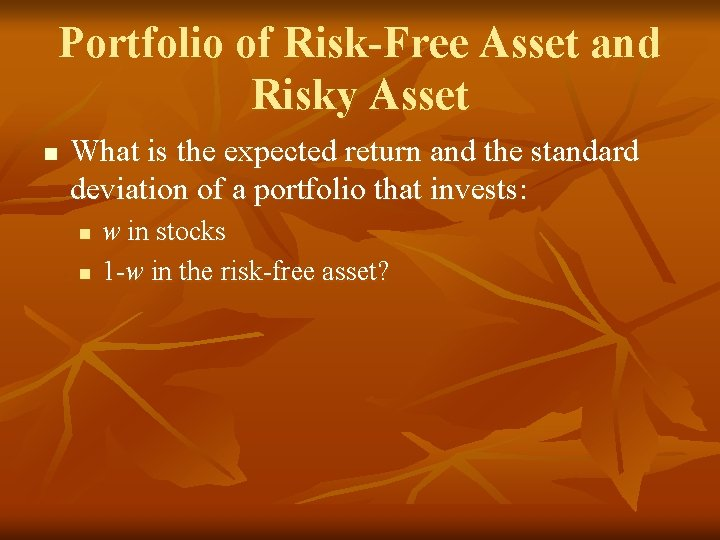Portfolio of Risk-Free Asset and Risky Asset n What is the expected return and the standard deviation of a portfolio that invests: n n w in stocks 1 -w in the risk-free asset?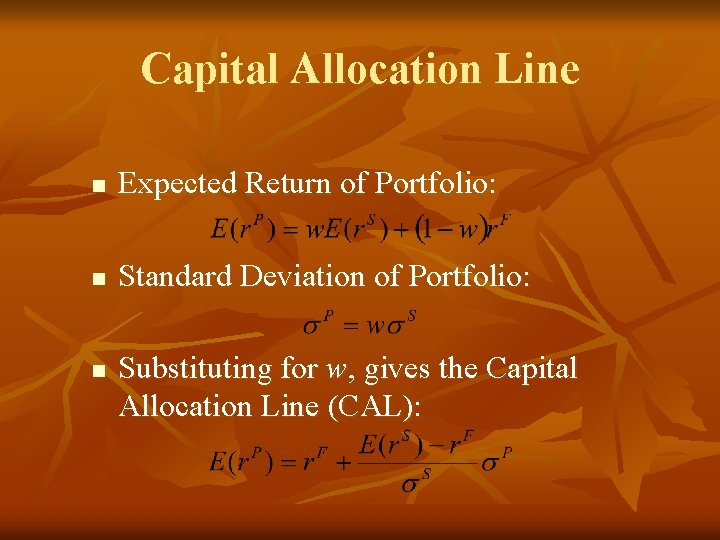Capital Allocation Line n Expected Return of Portfolio: n Standard Deviation of Portfolio: n Substituting for w, gives the Capital Allocation Line (CAL):Capital Allocation Line E(r) P rf 0 r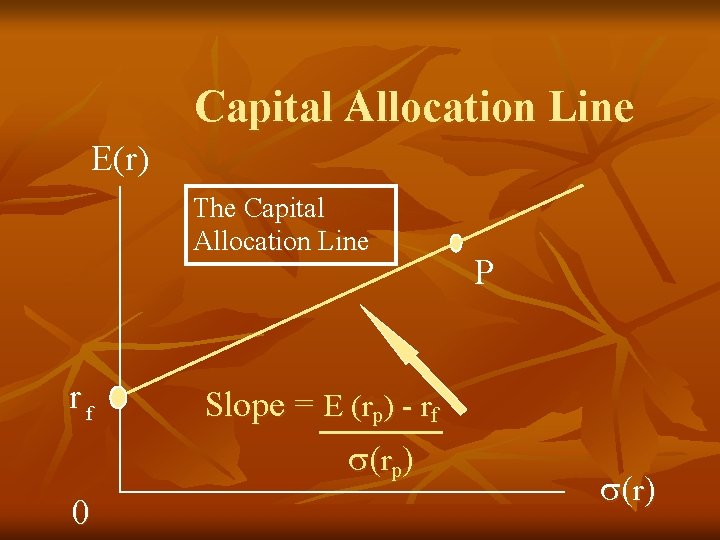Capital Allocation Line E(r) The Capital Allocation Line rf 0 Slope = E (rp) - rf rp P rCapital Allocation Line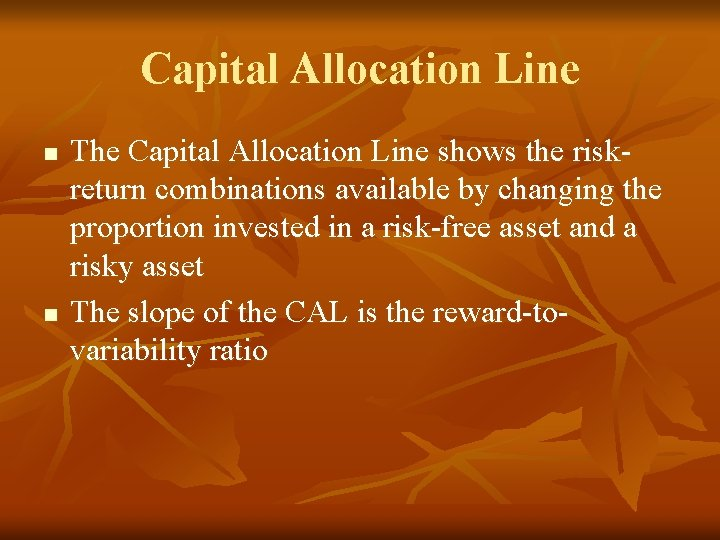Capital Allocation Line n n The Capital Allocation Line shows the riskreturn combinations available by changing the proportion invested in a risk-free asset and a risky asset The slope of the CAL is the reward-tovariability ratioCapital Assets Pricing Model n n n Now that we know that different stocks hold different levels of risk, we can use this knowledge to price the stocks. We use CAPM to find the rate at which to discount the future dividends of a stock. Perpetuity stock = D 1/k Growth stock = D 1/k-g Where D = dividend, g = growth, k = discount rate.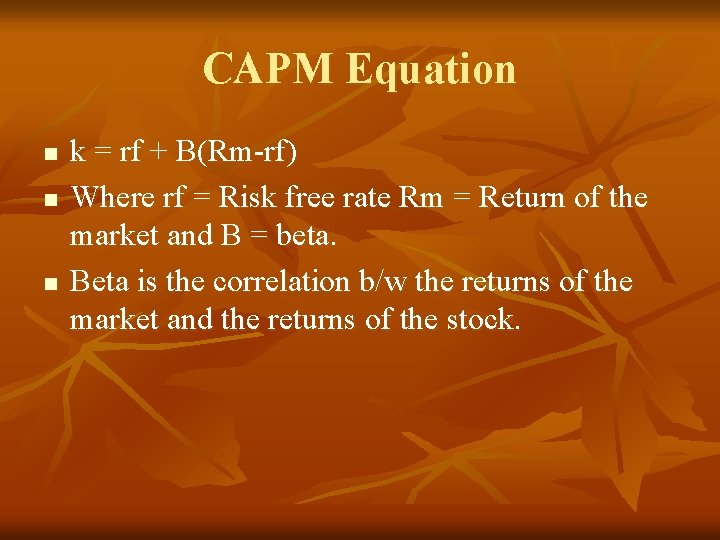CAPM Equation n k = rf + B(Rm-rf) Where rf = Risk free rate Rm = Return of the market and B = beta. Beta is the correlation b/w the returns of the market and the returns of the stock.R stock RMkt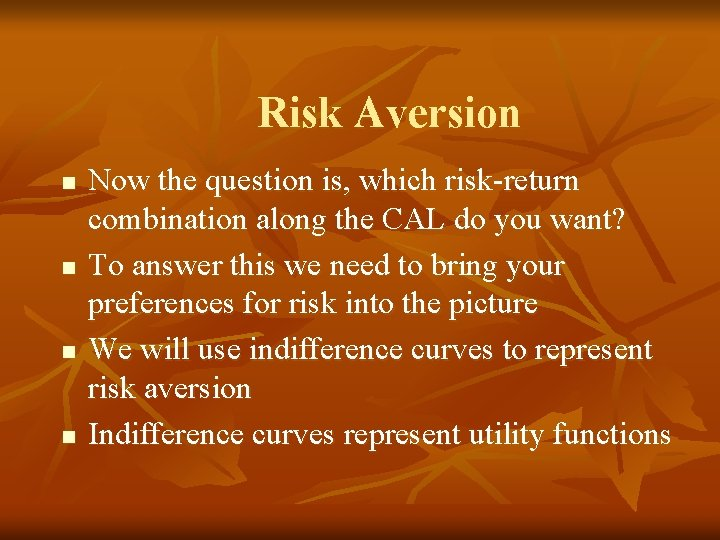Risk Aversion n n Now the question is, which risk-return combination along the CAL do you want? To answer this we need to bring your preferences for risk into the picture We will use indifference curves to represent risk aversion Indifference curves represent utility functions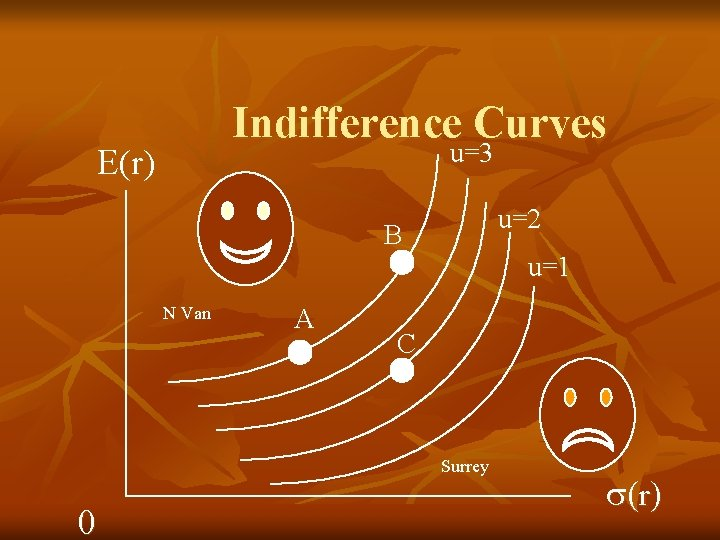Indifference Curves u=3 E(r) u=2 B N Van A u=1 C Surrey 0 rAsset Allocation n Now we can combine the indifference curves with the capital allocation line If investors are maximizing their utility, they will choose the highest possible indifference curve The highest curve is tangent to the CAL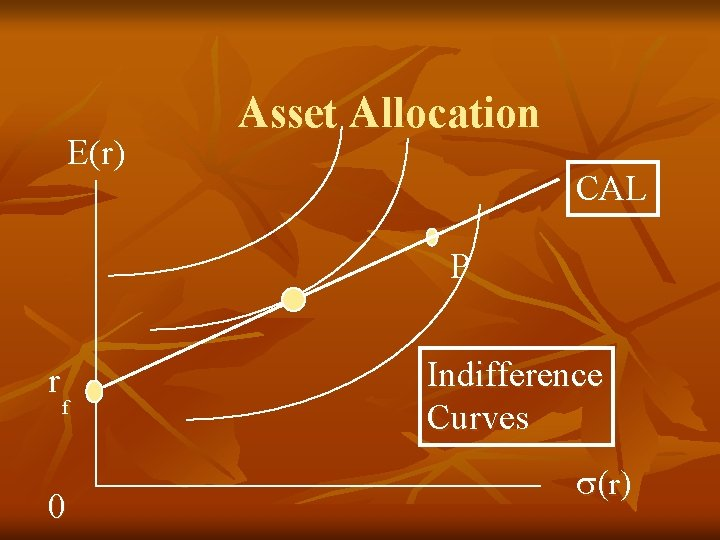E(r) Asset Allocation CAL P r f 0 Indifference Curves r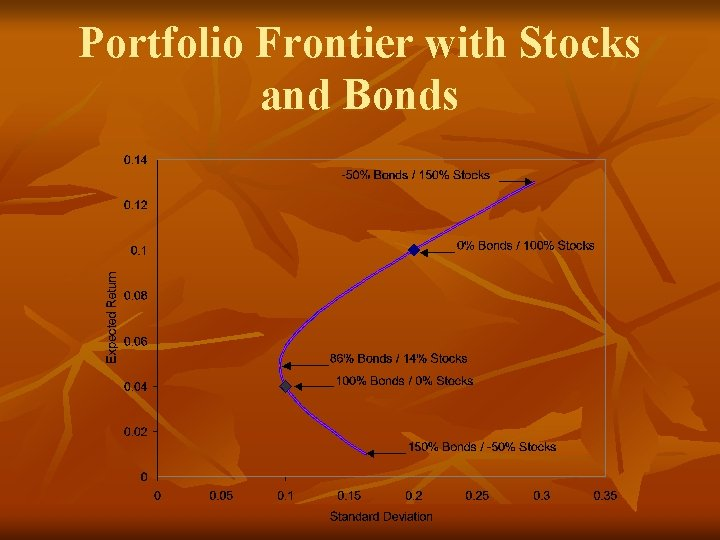Portfolio Frontier with Stocks and BondsPortfolio Frontier n n The portfolio frontier depicts the feasible portfolio choices for investors holding stocks and bonds The minimum variance portfolio includes 86% bonds and 14% stocks Portfolios below the minimum variance portfolio are inefficient The portfolio frontier above the minimum variance portfolio is called ‘efficient frontier’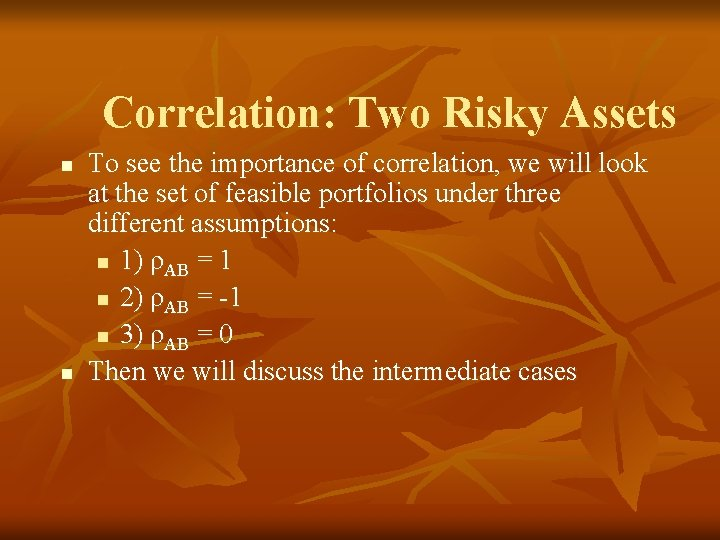Correlation: Two Risky Assets n n To see the importance of correlation, we will look at the set of feasible portfolios under three different assumptions: n 1) ρAB = 1 n 2) ρAB = -1 n 3) ρAB = 0 Then we will discuss the intermediate cases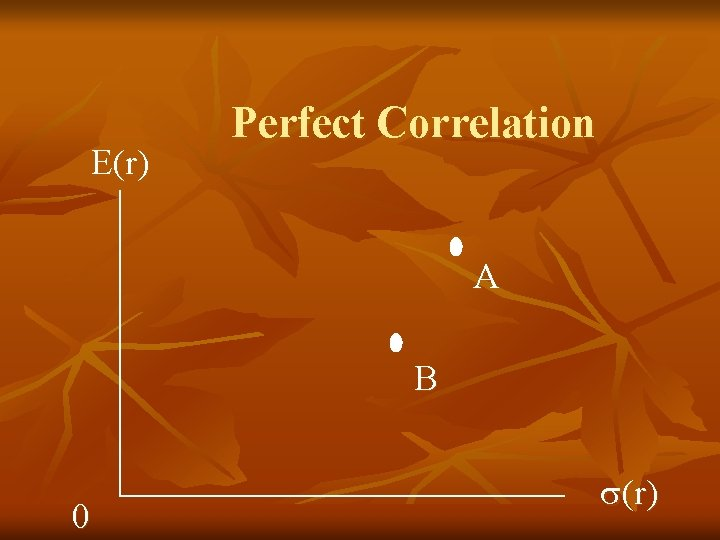E(r) Perfect Correlation A B 0 (r)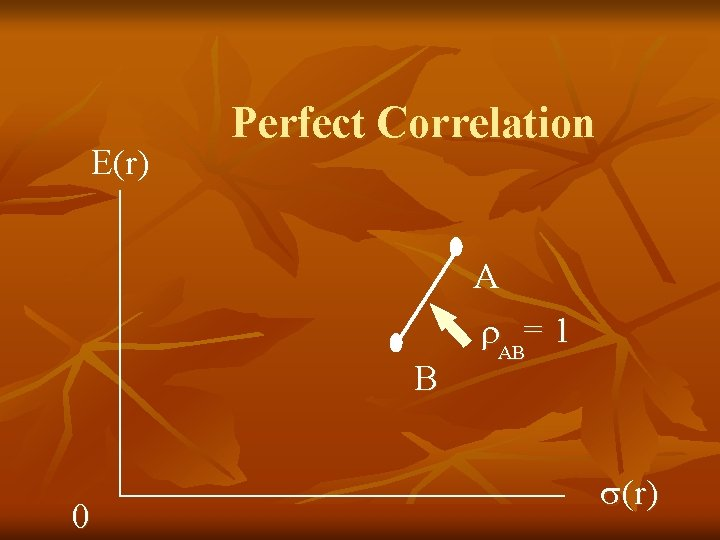E(r) Perfect Correlation B 0 A AB= 1 (r)Perfect Negative Correlation E(r) AB= -1 0 B A AB= 1 (r)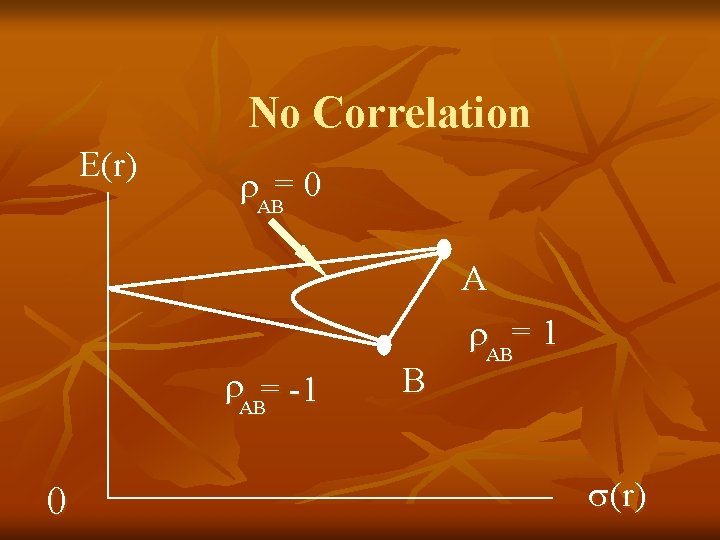No Correlation E(r) AB= 0 AB= -1 0 B A AB= 1 (r)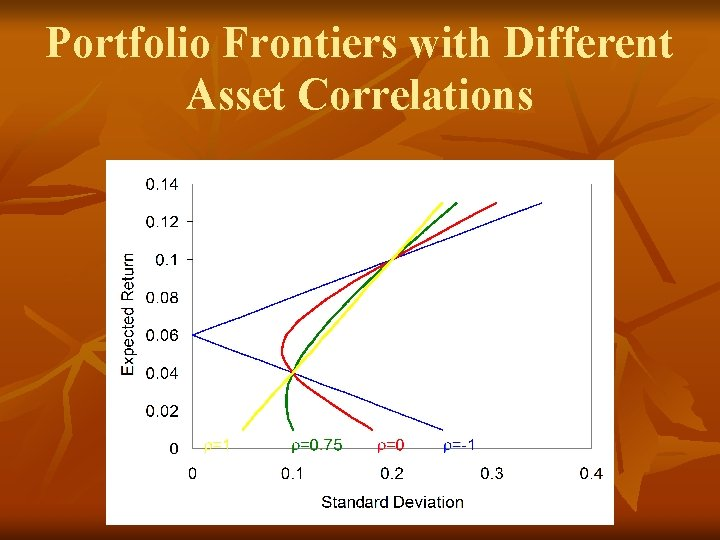Portfolio Frontiers with Different Asset Correlations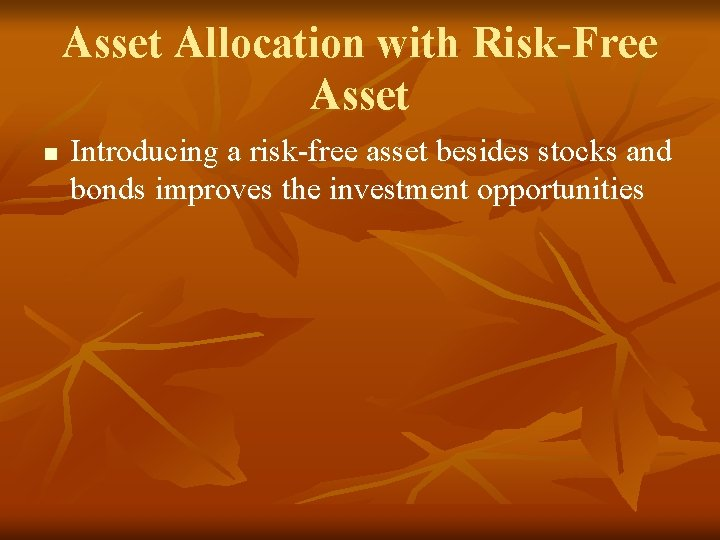Asset Allocation with Risk-Free Asset n Introducing a risk-free asset besides stocks and bonds improves the investment opportunitiesCapital Allocation Lines using Stocks and BondsOptimal Capital Allocation LinePortfolio Frontier with Three Risky Assets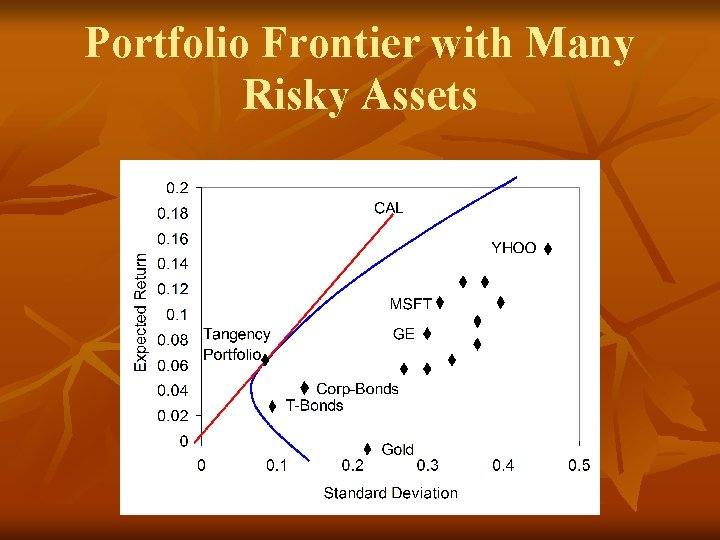Portfolio Frontier with Many Risky AssetsDiversification n n “It is part of a wise man … not to venture all his eggs in one basket” - Miguel de Cervantes “Put all your eggs in one basket and watch that basket” - Mark Twain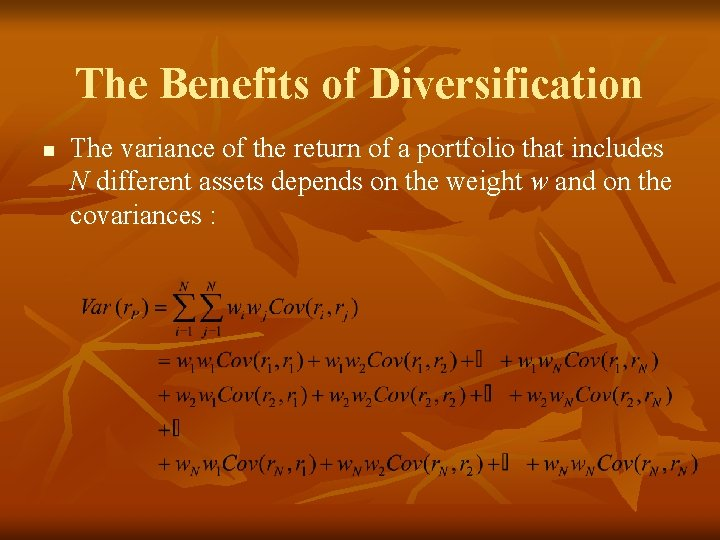The Benefits of Diversification n The variance of the return of a portfolio that includes N different assets depends on the weight w and on the covariances :Diversification n The standard deviation of a portfolio tends to decrease as more risky assets are added to the portfolio Std. Deviation Of Portfolio Firm-specific Risk Market Risk Number of SecuritiesQuestions?